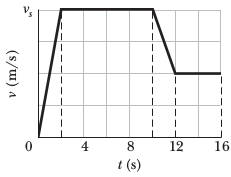# Problem: How far does the runner whose velocity-time graph is shown in the figure travel in 16 s? The vertical scaling is set by vs= 8.0 m/s.

###### FREE Expert Solution

We are asked to find the distance covered given velocity-time graph.

Anytime we're given a position, velocity, or acceleration graph to work with, a PVA diagram like the one below can help remind you of the relationships between the three functions.

$\mathbit{P}\begin{array}{c}{\mathbf{←}}\\ {\mathbf{\to }}\end{array}\underset{{\mathbit{s}}{\mathbit{l}}{\mathbit{o}}{\mathbit{p}}{\mathbit{e}}}{\overset{{\mathbit{a}}{\mathbit{r}}{\mathbit{e}}{\mathbit{a}}}{\mathbit{V}}}\begin{array}{c}{\mathbf{←}}\\ {\mathbf{\to }}\end{array}\mathbit{A}$

Moving left to right, we take the slope of the graph—the slope of a position graph is the velocity, and the slope of a velocity graph is the acceleration.

To move left to right, we use the area under the curve—but remember, that area only tells us the displacement or the change in velocity! To find a velocity, we take the area under an acceleration curve and add the initial velocity. To find a position, we'd take the area under a velocity curve and add the initial position

This problem is a little odd for its type, because we only want the displacement and not the final position. (Here, displacement is equal to distance traveled: since v ≥ 0 everywhere on the graph, the runner's velocity is always in the positive x-direction.)

81% (204 ratings)###### Problem Details

How far does the runner whose velocity-time graph is shown in the figure travel in 16 s? The vertical scaling is set by vs= 8.0 m/s.